# Poulet number

## Definition

A Poulet number or Sarrus number is an odd composite numbersuch that:.

In other words,divides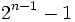. Equivalently,is a Fermat pseudoprime modulo.

## Occurrence

### Initial examples

The first few Poulet numbers are:

341, 561, 645, 1105, 1387, 1729, 1905, 2047, [SHOW MORE] View list on OEIS

These include, for instance:

• The Mersenne number, which is a Poulet number on account of the fact that Mersenne number for prime or Poulet implies prime or Poulet.
• Three Carmichael numbers -- these are numbers that are pseudoprime to every relatively prime base. These are.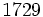is also known as the Hardy-Ramanujan number, and is the smallest number expressible as the sum of two cubes in two distinct ways.

### Infinitude

Further information: Infinitude of Poulet numbers

There are infinitely many Poulet numbers. This can be proved in many ways. For instance, Mersenne number for prime or Poulet implies prime or Poulet. This shows that if we find one Poulet number, we can iterate the operation of taking the Mersenne number and obtain infinitely many Poulet numbers.

## Facts

Statement Kind of numbers it says are Poulet numbers Smallest example Proof idea
Mersenne number for prime or Poulet implies prime or Poulet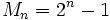whereitself is a Poulet number OR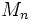whereis prime andisn't 2047 (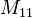) factors as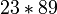Use thatdividesas a polynomial with integer coefficients.
Composite Fermat number implies Poulet numberwhere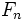is not prime 4294967297 (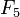) factors as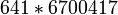Use thatdividesas a polynomial with integer coefficients, and also that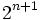divides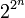because.
Square of Wieferich prime is Poulet number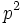whereis a prime such that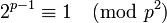1194649 (square of 1093) Use thatdividesas a polynomial with integer coefficients twice (in base, then in exponent)
Product of successive primes in Cunningham chain of the second kind satisfying congruence conditions is Poulet numberwhere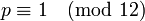and bothare primes 2701 (product of 37 and 73) apply Fermat's little theorem, condition for 2 to be a quadratic residue
Infinitude of Poulet numbers N/A N/A Use the Mersenne number iteratively, after having found at least one Poulet number.